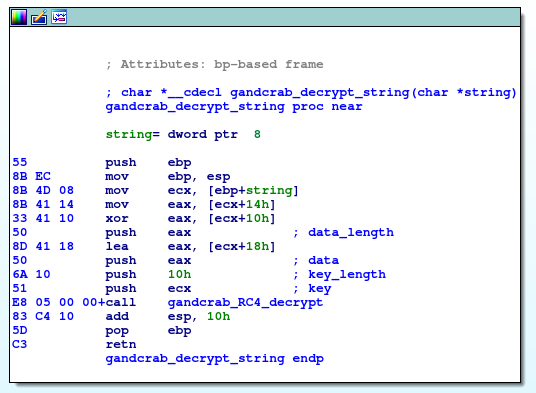## Introduction

In the post about GandCrab String Decryption I use very simple heuristic for identifying the function for string decryption. Because this kind of funtion is usually heavily used, I made an assumption that the scting decryption function is the most used function in our sample. This assumption is correct for GandCrab v5.1 DLL files, but it turns out that it is not true for GandCrab v5.2 and v.53. EXE samples.

One quick fix can be trying for example 3 (or maybe 5) most used functions as the string decryption function, but it is not clear solution and also it can be the wrong assumption.in some cases. So, it is time to rewrite algorithm for finding the string decryption function.

## Find the correct GandCrab string decryption function

First, remind the structure of GandCrab string decryption function. Let’s review its disassembly in the following picture:The assumption that this function is heavily used is true, but it is not necessary that this function is the most used function. In reviewed samples this function is called 185, 158, 174 and 158 times. So, let’s assume that this function is called at least 100-times.

Next, the only purpose of this function is preparing parameters for RC4 decryption from input argument, so this function should be short. It seems that the length of this function is less then 0x20 bytes, so it should be safe to assume that length should be less then 0x25 bytes.

Finally, the string decryption function makes exactly one call (to RC4 decryption) and prepares 4 parameters for it (4 pushes instruction). Together with one push from the prologue this function should contain 5 pushes and 1 call instruction. With these 4 assumptions (length, count of xrefs, count of pushes and count of calls) it sould be possible to find string decryption function. Another approach can be to identify RC4 decryption routine and than find the functions from which is RC4 decryption referenced.

My script modified in the way described above workd with all tested samples (GandCrab v5.1, v5.2, v5.3, DLL and EXE files). The updated alorithm for finding decryption function now looks like this:

``````static find_decrypt_function() {
// find the address of the string decryption function with following conditions:
//   it is short (up to 0x25 bytes)
//   it is heavily used (at least 100 xrefs)
//   it contains exactly one call instruction (for calling RC4 decryption routine)
//   it contains exactly 5 push instructions (one push ebp from prologue and 4 arguments for RC4)
auto func_start, func_end, found;
found = 0;
func_start = NextFunction(0);
func_start = NextFunction(func_start);
func_end = FindFuncEnd(func_start);
if (func_end-func_start < 0x25) {
push_count = 0;
call_count = 0;
if (GetMnem(addr) == "push") push_count = push_count + 1;
if (GetMnem(addr) == "call") call_count = call_count + 1;
}

if ((push_count == 5) && (call_count == 1) && (count_xrefs(func_start) > 100))  {
found = 1;
}
}
//Message("%08x: %08x, %d --> %d\n", func_start, func_end, count_xrefs(func_start), found);
}

return func_start;
}
``````

## Credits

Thanks to Marcelo Rivero for reporting the issue with GandCrab v5.2 and v5.3 EXE files, sharing the samples and testing.